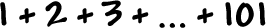Do you think we can find a formula that will work for adding all the integers from 1 to n?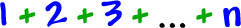Think about it!  Look over the last three problems we just did -- see anything?

Let's write it out the same way: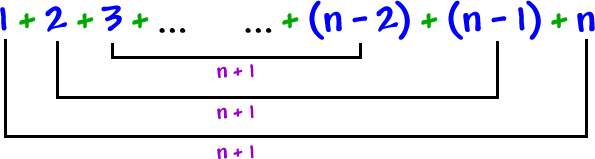How many pairs of n + 1 are there?  Half of n!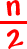pairs of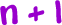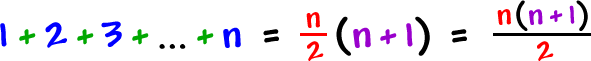TEST IT:

Find the sum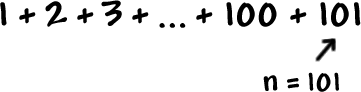(You know it will be 5050 + 101 = 5151.)

Does the formula work?

How would you write this series in sigma notation?